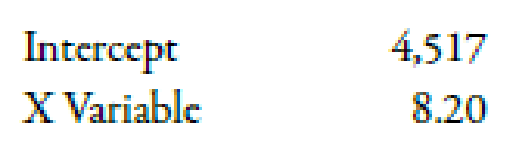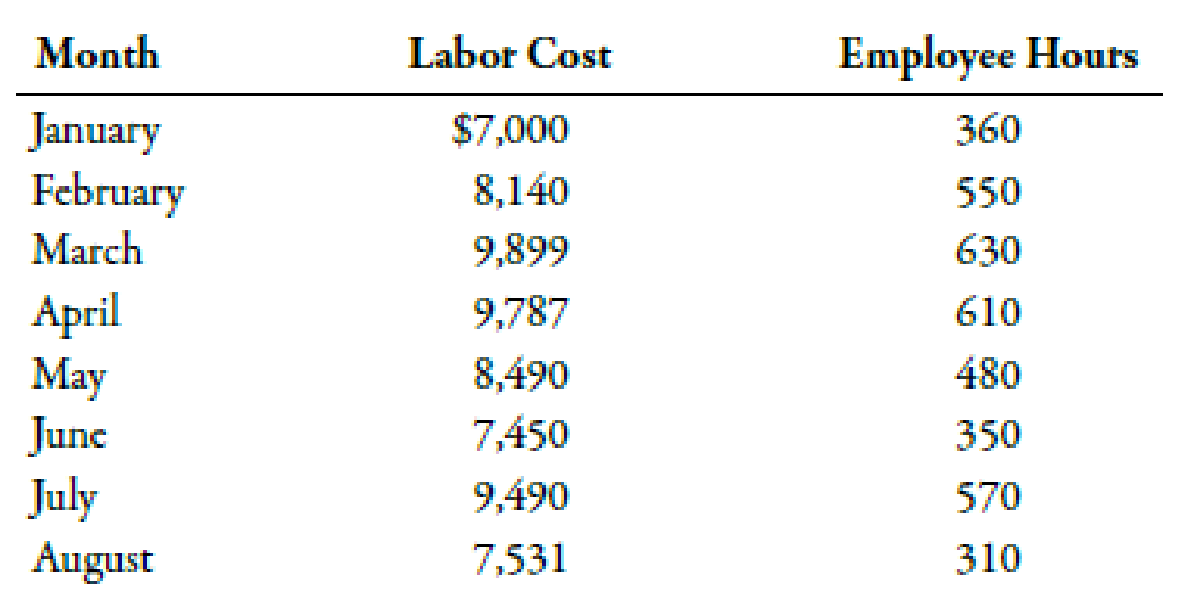Chapter 3, Problem 20BEA### Managerial Accounting: The Corners...

7th Edition
Maryanne M. Mowen + 2 others
ISBN: 9781337115773

#### Solutions

Chapter
Section### Managerial Accounting: The Corners...

7th Edition
Maryanne M. Mowen + 2 others
ISBN: 9781337115773
Textbook Problem
1 views

# Using Regression to Calculate Fixed Cost, Calculate the Variable Rate, Construct a Cost Formula, and Determine Budgeted CostRefer to the information for Pizza Vesuvio on the previous page. Coefficients shown by a regression program for Pizza Vesuvio’s data are:Required:Use the results of regression to make the following calculations: 1. Calculate the fixed cost of labor and the variable rate per employee hour. 2. Construct the cost formula for total labor cost. 3. Calculate the budgeted cost for next month, assuming that 675 employee hours are budgeted. (Note: Round answers to the nearest dollar.) Use the following information for Brief Exercises 3-17 through 3-20:Pizza Vesuvio makes specialty pizzas. Data for the past 8 months were collected:1.

To determine

Compute the value of labor fixed cost and the variable rate per employee hour.

Explanation

Cost:

Cost can be defined as the cash and cash equivalent which is incurred against the products or its related services which will benefit the organization in the future. There are two types of costs that are fixed and variable costs.

Fixed cost of labor is \$4,517...

2.

To determine

Prepare the cost formula for total labor cost.

3.

To determine

Compute the value of budgeted labor costs for next month, if 675 employee hours are budgeted.

### Still sussing out bartleby?

Check out a sample textbook solution.

See a sample solution

#### The Solution to Your Study Problems

Bartleby provides explanations to thousands of textbook problems written by our experts, many with advanced degrees!

Get Started